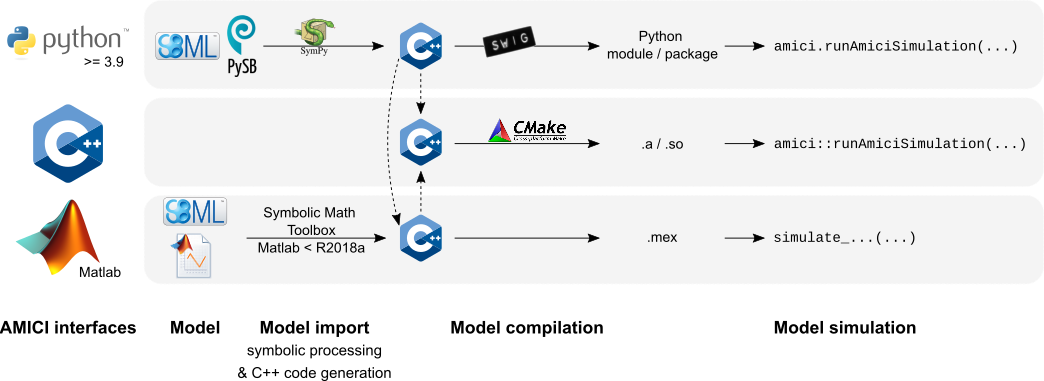AMICI provides a multi-language (Python, C++, Matlab) interface to the SUNDIALS solvers CVODES (for ODEs) and IDAS (for DAEs). AMICI allows the user to read differential equation models specified as SBML or PySB and automatically compiles such models into Python modules, C++ libraries or .mex simulation files (Matlab).

In contrast to the (no longer maintained) sundialsTB Matlab interface, all necessary functions are transformed into native C++ code, which allows for a significantly faster simulation.

Beyond forward integration, the compiled simulation file also allows for forward sensitivity analysis, steady state sensitivity analysis and adjoint sensitivity analysis for likelihood-based output functions.

The interface was designed to provide routines for efficient gradient computation in parameter estimation of biochemical reaction models but it is also applicable to a wider range of differential equation constrained optimization problems.

## Features

• SBML import

• PySB import

• Generation of C++ code for model simulation and sensitivity computation

• Python, C++, Matlab interface

• Sensitivity analysis

• forward

• first- and second-order (second-order Matlab-only)

• Pre-equilibration and pre-simulation conditions

• Support for discrete events and logical operations

## Interfaces & workflow

The AMICI workflow starts with importing a model from either SBML (Matlab, Python), PySB (Python), or a Matlab definition of the model (Matlab-only). From this input, all equations for model simulation are derived symbolically and C++ code is generated. This code is then compiled into a C++ library, a Python module, or a Matlab .mex file and is then used for model simulation.The functionality of the Python, Matlab and C++ interfaces slightly differ, as shown in the following table:

Feature \ Interface

Python

C++

Matlab

SBML import

yes (details)

no

yes (<=R2017b)

PySB import

yes

no

no

DAE import

no

no

yes

Forward sensitivities

yes

yes

yes

yes

yes

yes

yes

yes

yes

Second-order sensitivities

no

no

yes

Events

yes

yes

yes

preequilibration

yes

yes

yes

presimulation

yes

yes

no